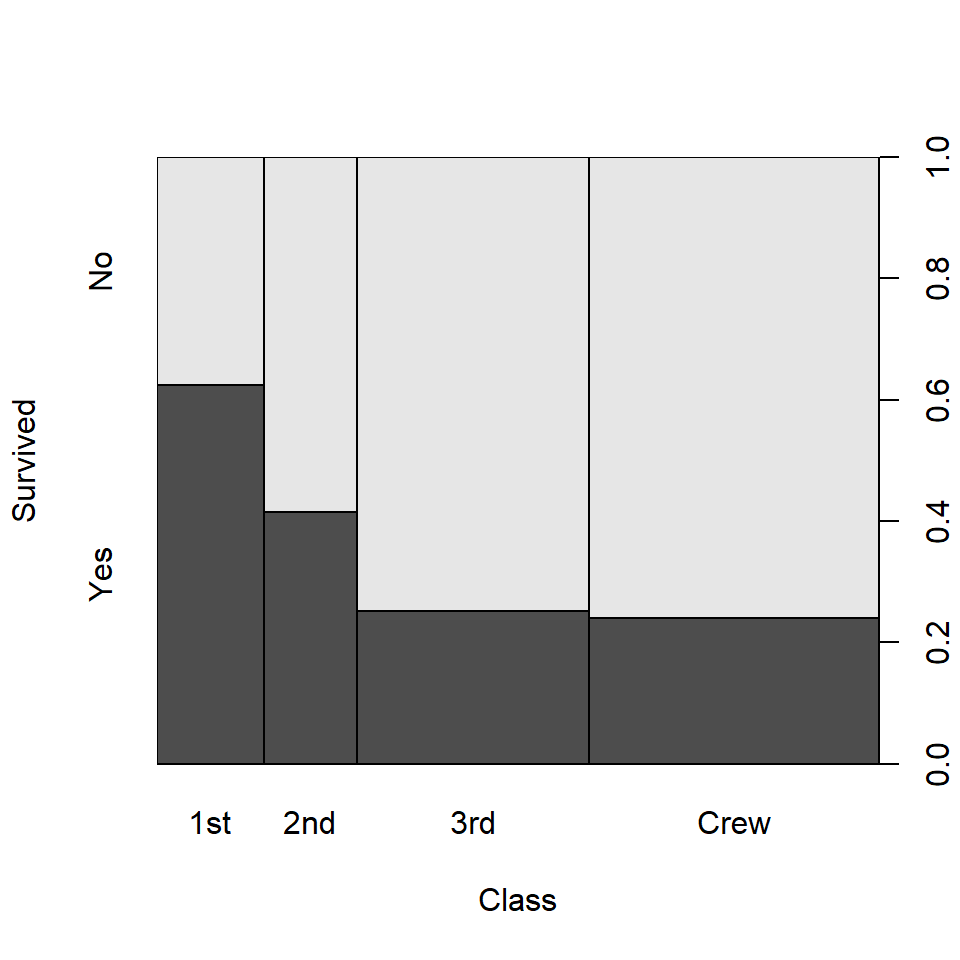# Spineplot in R

## Data

The following table contains the count the number of Titanic survivals based of their economic status (class). This data will be used in the examples below.

``````# Data
dat <- apply(Titanic, c(1, 4), sum)``````
class \ Survived No Yes
1st 122 203
2nd 167 118
3rd 528 178
Crew 673 212

## Spineplot

Spineplots are a generalization of stacked bar charts. The `spineplot` function allows creating this type of graph in base R (actually a mosaic plot, which is a spineplot for two or more variables). See `?spineplot` to know more about the possible input options.

``spineplot(dat)``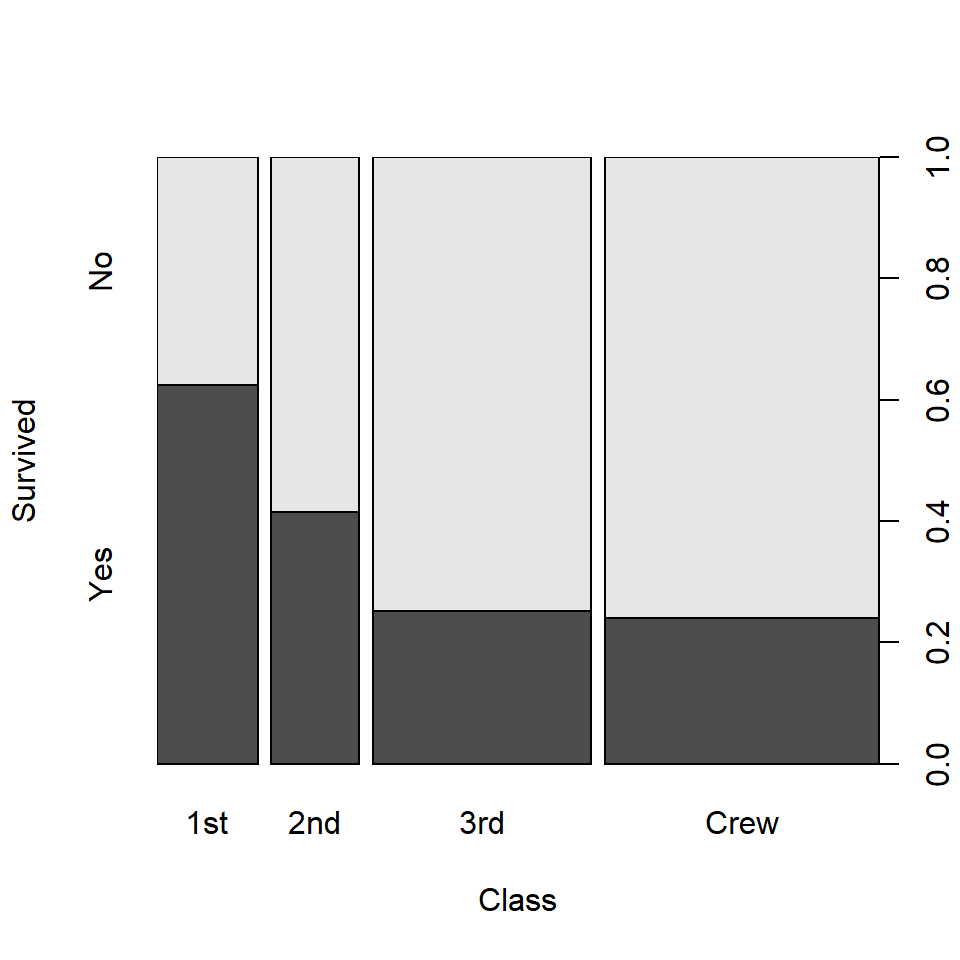Transpose the variables

The spineplot can be flipped transposing the data, as in the example below.

``spineplot(t(dat))``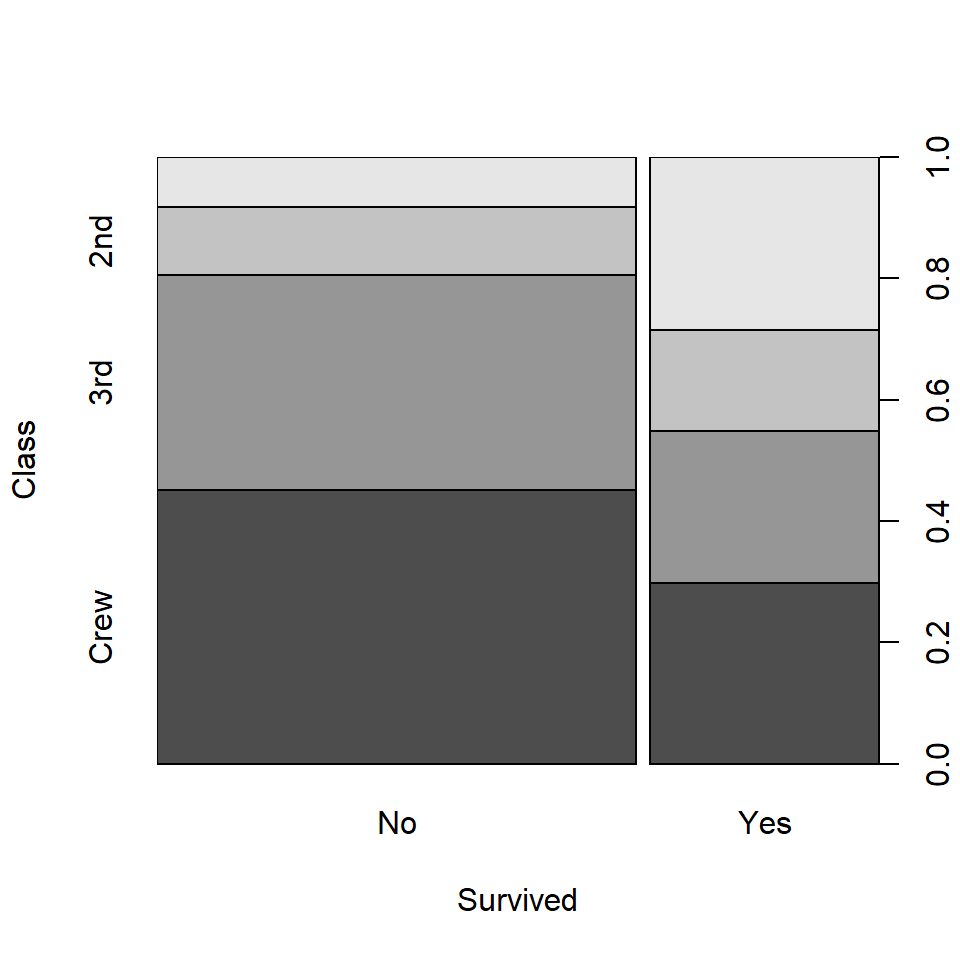### Color customization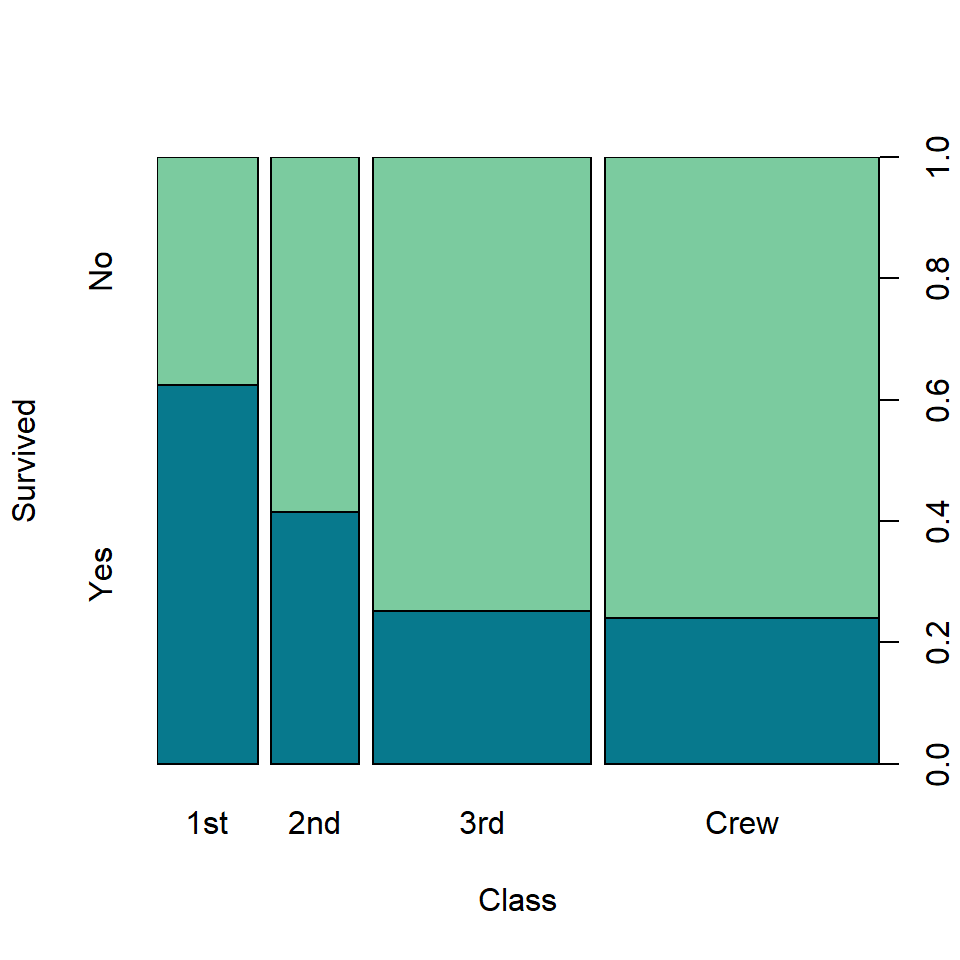Fill color

You can pass a vector of colors to `col` to customize the default gray scale colors. Pass as many colors as classes on the Y-axis.

``spineplot(dat, col = c("#07798D", "#7BCB9F"))``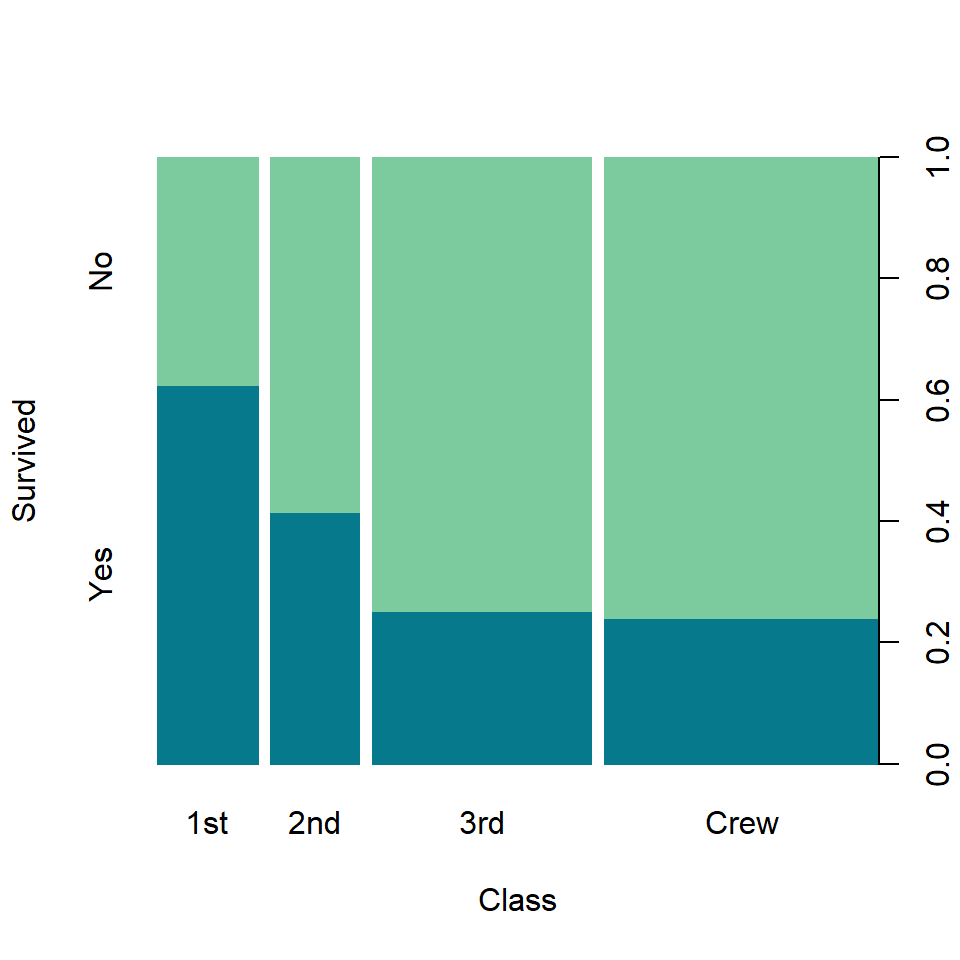Border color

You can also customize the border color with `border`. In this example we are setting it to the fill color.

``````spineplot(dat, col = c("#07798D", "#7BCB9F"),
border = c("#07798D", "#7BCB9F"))``````

## Spinogram

Spinogram

Spinograms are a generalization of histograms. The default `spineplot` function creates a spineplot, but setting `off = 0` a spinogram will be created, removing the offset between the bars.

``spineplot(dat, off = 0)``